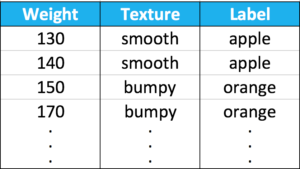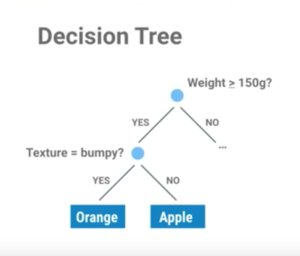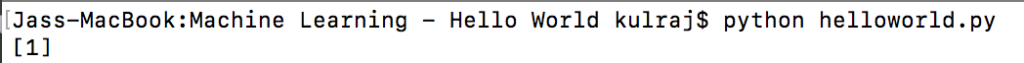# Kulraj Suri

Software Engineer / Quant Dev

# Introduction

In this post, we’ll attempt to write our first machine learning program in Python.

Specifically, we’ll create a machine learning program that predicts whether an input is an apple or an orange – this is classification, a form of supervised machine learning.

Machine learning is the study of algorithms that learn from examples and experience instead of relying on hard coded rules.

Here, we’re going to train a classifier. We can think of a classifier as a function which takes some data as input and assigns a label to it as output.

# RecipeWe’ll follow the simple recipe of:

• Collect the training data
• These are examples of the problem we want to solve
• Train the classifier
• For our problem, we are going to write a function to classify a type of fruit, specifically an apple or orange
• Make predictions
• It will take description of the fruit as input and predict whether it is an apple or orange as output based on features like its weight and texture

# Collect the Training DataFirst we’ll collect data about apples and oranges, specifically measurements such as their weight & texture and label them accordingly.

In machine learning, these measurements are called features. We’ll use just two to keep things simple. A good feature makes it easy to discriminate between different types of fruit.

The last column in each row is called the label. It identifies what type of fruit is in each row and there are just two possibilities: apples and oranges.

Each row in the training data is an example. It describes one piece of fruit.

Now, lets write down out training data in code. We’ll use two variables: features and labels. Features contain the first two columns and labels contain the last.

• We can think of features as inputs to the classifier and labels as the output we want.Modifying Training Data

Scikit-learn uses real-valued features so we change out variable types to ints instead of strings.

• We’ll use 0 for bumpy and 1 for smooth
• Similarly we’ll use 0 for apple and 1 for orange# Train Classifier

The type of classifier we’re going to use is called a decision tree:• For now, we’ll think of a classifier as a set of rules
• There are many different types of classification algorithms but the input and output type is always the same

On line 1, we’ll import the decision tree algorithm from the machine learning module sklearn.Following that, we’ll create the classifier. At this point it’s just an empty set of rules. It doesn’t know anything about apples or oranges yet.To train it, we’ll need a learning algorithm.

• If a classifier is a set of rules then we can think of a learning algorithm as the procedure that creates them
• It does this by finding patterns in the training data

In scikit, the training algorithm is included in the classifier object and is called fit:# Make Predictions

At this point, we have a trained classifier that we can use to make predictions on the type of fruit.

The input to the classifier is the features of our new example.

• Weight = 160 grams
• Texture = bumpyThe output will be 0 if it is an apple or 1 if it is an orange.

• We can clearly guess that our example is an orange.

When we run the program, we can see that it classified our new example as an orange.